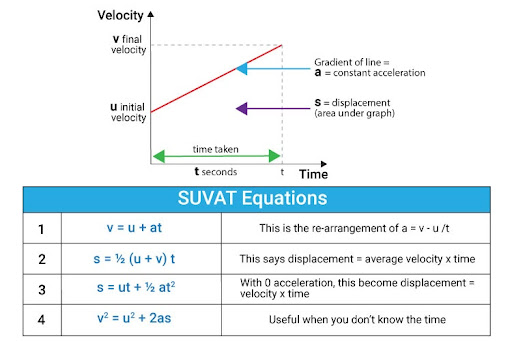# What are the SUVAT equations and how would you apply them to a question?

What are the SUVAT equations and how would you apply them to a question?
SUVAT equations are kinematic equations used when the velocity is not constant, i.e., uniform (constant acceleration). The acceleration must be constant. S stands for displacement, U stands for initial velocity, V stands for final velocity, A stands for acceleration, and T stands for time. There are 5 SUVAT equations that relate 4 of the variables together. The SUVAT equations can be derived from acceleration, i.e., change in velocity / time or a = v - u /t or v = u + at.
• Equation 1 : v = u +at
• Equation 2: s = ut + ½ at^2
• Equation 3: s = ½ (u + v) t
• Equation 4: v^2 = u^2 + 2as
• Equation 5: s = vt - ½ at^2
To use these equations, write the variables you are given and the unknown variable and choose the equation which relates to these variables. For example, equation 1 can be used to calculate v, u, a, or t. Equations 2, 3, 4, 5 also relate displacement.
As the acceleration is constant, the third SUVAT equation is derived from the average speed. Equation 1 (with t as subject) can be substituted in equation 3 to derive equation 4. Equation 1 can also be substituted directly in equation 3 to derive equation 2 and equation 1 (with u subject) can be substituted into equation 4  to derive equation 5.
To use SUVAT equations, choose the equation which relates the known and unknown variables.